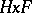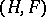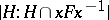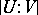A pair of subgroupsof a groupwhich are members of the decomposition ofinto double cosets, i.e. in the decomposition ofinto non-intersecting subsets of the type, whereis an element of. A subsetis said to be a coset of the groupmoduloor a double coset of the groupmodulo. Thus, the decomposition of a group of order 24 into double cosets modulo, whereandare its Sylow- and-subgroups, consists of a single coset modulo. Any double cosetconsists ofcosets ofbyand, at the same time, ofcosets ofby, wheredenotes the index of a subgroupin a group.